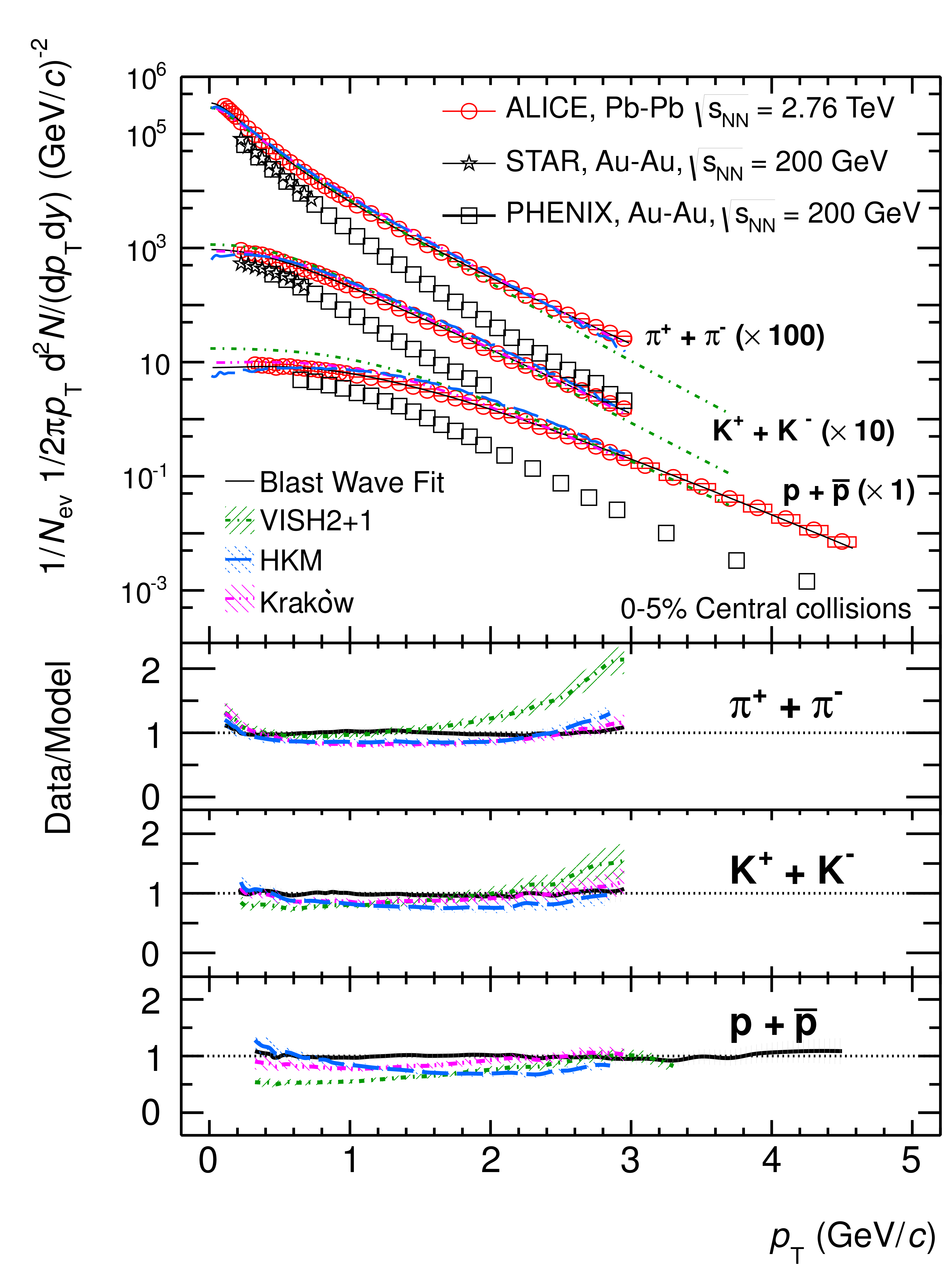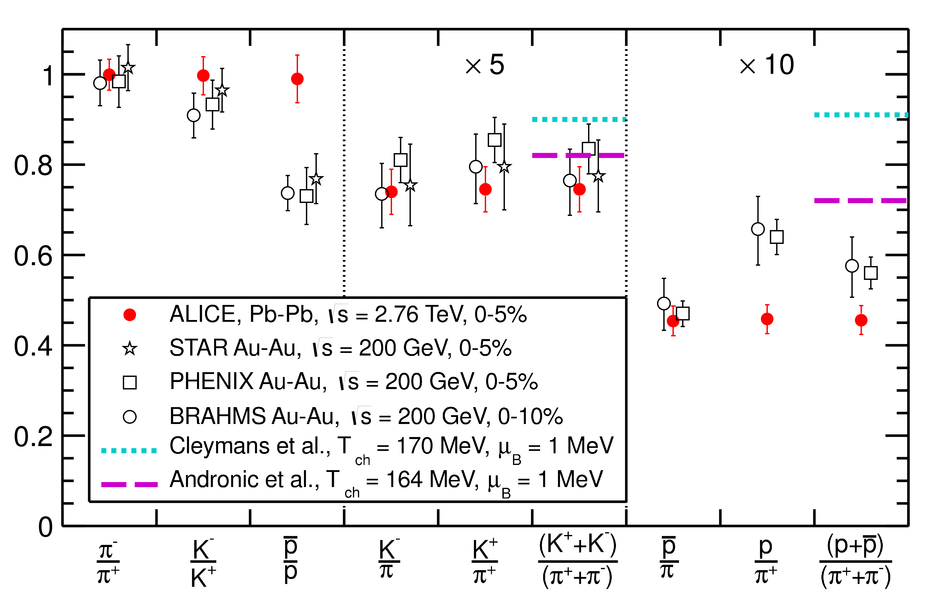# Pion, Kaon, and Proton Production in Central Pb--Pb Collisions at $\sqrt{s_{NN}} = 2.76$ TeV

In this Letter we report the first results on $\pi^\pm$, K$^\pm$, p and pbar production at mid-rapidity (|y|<~0.5) in central Pb-Pb collisions at sqrt{s_{NN}} = 2.76 TeV, measured by the ALICE experiment at the LHC. The pT distributions and yields are compared to previous results at sqrt{s_{NN}} = 200 GeV and expectations from hydrodynamic and thermal models. The spectral shapes indicate a strong increase of the radial flow velocity with sqrt{s_{NN}}, which in hydrodynamic models is expected as a consequence of the increasing particle density. While the K/pi ratio is in line with predictions from the thermal model, the p/pi ratio is found to be lower by a factor of about 1.5. This deviation from thermal model expectations is still to be understood.

Figures

## Figure 1

 Transverse momentum distributions of the sum of positive and negative particles (box: systematic errors; statistical errors smaller than the symbol for most data points), fitted individually with a blast wave function, compared to RHIC data and hydrodynamic models.## Figure 2

 Mid-rapidity particle ratios, compared to RHIC results~ and predictions from thermal models~ for central Pb-Pb collisions at the LHC (combined statistical and systematic errors).